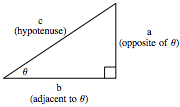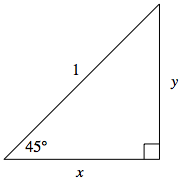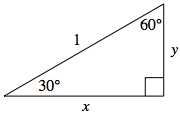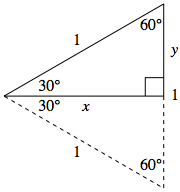## Right Triangle Trigonometry

Recall from geometry that given two similar triangles, the ratio of two sides in one triangle will be identical to the ratio of the two corresponding sides in the other triangle.

Note also that any two right triangles that both contain an angle $\theta \lt 90^{\circ}$ must be similar, as the three angles for each must then be $90^{\circ}$, $\theta$, and $90^{\circ} - \theta$.

This means that for any angle $\theta$, we can consider any right triangle that contains that angle $\theta$, and the ratios of side lengths seen will always be the same.

Suppose we draw such a triangle, labeling it as shown below:Consequently, $a/b$, $a/c$, and $b/c$ will be constants that depend only on the value of $\theta$ -- the actual length of any particular side doesn't matter.

Given this dependency on $\theta$, one should find it natural to define functions that yield these proportions. We define them below:

$$\begin{array}{rcccl} \sin \theta &=& \frac{a}{c} &=& \frac{opp}{hyp}\\\\ \cos \theta &=& \frac{b}{c} &=& \frac{adj}{hyp}\\\\ \tan \theta &=& \frac{a}{b} &=& \frac{opp}{adj}\\\\ \cot \theta &=& \frac{b}{a} &=& \frac{adj}{opp}\\\\ \sec \theta &=& \frac{c}{b} &=& \frac{hyp}{adj}\\\\ \csc \theta &=& \frac{c}{a} &=& \frac{hyp}{opp} \end{array}$$

We call these functions sine, cosine, tangent, cotangent, secant, cosecant, respectively.

Notice as shown above, we often write these functions without the traditional parentheses around the function's input, when the input to be used is clear -- but one should treat such expressions as if the parentheses were actually there. (e.g., $\sin x = \sin (x)$, $\cos x = \cos (x)$, etc...)

To aid in computing one of these functions for a particular value of $\theta$ given another, one should note two things:

First, we know by the Pythagorean Theorem, we know $a^2+b^2=c^2$.

Dividing each term by $c^2$ gives $\displaystyle{ \frac{a^2}{c^2} + \frac{b^2}{c^2} = 1}$, or equivalently

$$\sin^2 \theta + \cos^2 \theta = 1$$

The above is a fundamental identity when dealing with trigonometry -- one would be well-served by committing it to memory!

Also, the six functions defined above occur in reciprocal pairs:

$$\begin{array}{rcl} \csc \theta &=& \frac{1}{\sin \theta}\\\\ \sec \theta &=& \frac{1}{\cos \theta}\\\\ \cot \theta &=& \frac{1}{\tan \theta} \end{array}$$

### Values of the Sine and Cosine Functions for Special Angles

For certain angles, we can appeal to geometry to find the exact values of the sine and cosine functions.Consider a $45^{\circ}$ angle, as shown in the triangle below. Any hypotenuse length will yield the coming conclusion, so we choose a convenient length of $1$. Recall, since the sum of angles in a triangle is $180^{\circ}$, the unmarked angle must also measure $45^{\circ}$. With two angles of the same measure, geometry tells us that the triangle is isosceles, with lengths $x$ and $y$ equal.

Then, the Pythagorean Theorem gives us:

$$\begin{array}{rcll} x^2 + y^2 &=& 1 & \scriptsize{\textrm{since } x = y, \textrm{we make a substitution}}\\ x^2 + x^2 &=& 1 & \scriptsize{\textrm{now we solve for } x}\\ 2x^2 &=& 1 &\\ x &=& \frac{\sqrt{2}}{2} &\scriptsize{\textrm{keeping only the positive solution, as } x \textrm{ represents a length}}\\ y &=& \frac{\sqrt{2}}{2} &\scriptsize{\textrm{again, recalling } x = y}\\ \end{array}$$

Now knowing the lengths of the hypotenuse and the sides adjacent to and opposite of the $45^{\circ}$ angle, we can deduce

$$\begin{array}{rcl} \cos 45^{\circ} &=& \frac{\sqrt{2}}{2}\\\\ \sin 45^{\circ} &=& \frac{\sqrt{2}}{2} \end{array}$$

We may similarly use geometry to find the values of the sine and cosine functions when applied to angles measuring $30^{\circ}$ and $60^{\circ}$.

This time we start with a $30^{\circ}-60^{\circ}-90^{\circ}$ right triangle whose hypotenuse is again one unit in length, as shown on the right.

Note, if we construct a congruent $30^{\circ}-60^{\circ}-90^{\circ}$ triangle directly below this one, as shown below, we form a larger triangle whose angle measures are all $60^{\circ}$.

This larger triangle must then be equilateral, with all sides of length one, forcing the value of $y$ to be half that.

Again appealing to the Pythagorean theorem, we now have:

$$\begin{array}{rcll} x^2 + y^2 &=& 1 & \scriptsize{\textrm{then we substitute } y = \frac{1}{2}}\\ x^2 + \left(\frac{1}{2}\right)^2 &=& 1 & \scriptsize{\textrm{from here we can solve for } x}\\ 4x^2 + 1 &=& 4 &\\ x &=& \frac{\sqrt{3}}{2} & \overset{\normalsize{\textrm{again keeping only the positive value}}}{\scriptsize{\textrm{ of } x \textrm{ as it represents a length}}}\\ \end{array}$$Now we know the lengths of the hypotenuse and the sides adjacent to and opposite both the $30^{\circ}$ and the $60^{\circ}$ angles. Using the definitions of $\sin t$ and $\cos t$ above, we quickly discover

$$\begin{array}{rcl} \cos 30^{\circ} &=& \frac{\sqrt{3}}{2}\\\\ \sin 30^{\circ} &=& \frac{1}{2}\\\\ \cos 60^{\circ} &=& \frac{1}{2}\\\\ \sin 60^{\circ} &=& \frac{\sqrt{3}}{2}\\\\ \end{array}$$